login  home  contents  what's new  discussion  bug reports help  links  subscribe  changes  refresh  edit

## Simplification of Expressions

Suppose we compute

fricas
integrate(exp(-x^2/2)/sqrt(2*%pi),x=%minusInfinity..%plusInfinity)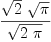(1)
Type: Union(f1: OrderedCompletion?(Expression(Integer)),...)

And now I wonder why common factors are not cancelled and why not the result "1" is produced.

In general (unlike some other computer math systems) FriCAS automatically performs only a very small number of basic simplifications. This is not one of them, so we need to provide some help. In particular we need to tell FriCAS how to expand square roots. (Since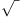is a multi-valued function this rule is true only in a restricted sense for a particular choice of branches. Consider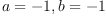.)

fricas
expandSqrt := rule sqrt(a*b)==sqrt(a)*sqrt(b)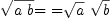(2)
Type: RewriteRule?(Integer,Integer,Expression(Integer))

Next, notice that the output of the integration operation has a complicated type structure. This would interfere with the simplification, so we first simplify the type before we apply the rule.

fricas
(%% 1)::Expression Integer(3)
Type: Expression(Integer)
fricas
expandSqrt %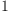(4)
Type: Expression(Integer)

More generally, most powerful simplifications are done by normalize, but currently normalize performs only minimal transformations on roots. Also, variant of normalize is run on the input to the integrator, but not on the output. For best result we should normalize output (but that is tricky, because we want to preserve structure of the input in the output).

 Subject:   Be Bold !! ( 14 subscribers )